# Ridge Regression

Online Ridge Regression is the application of the regularized Least Squares method to the online linear regression setting.

The algorithm works as follows (all the vectors are columns):

Initialize vector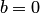, and matrix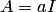;
FORRead new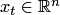Output prediction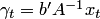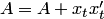Read new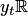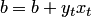END FOR.

The Aggregating Algorithm Regression (AAR) almost repeats RR, with the difference that in AAR the matrix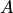is updated before the prediction has made. Upper bounds for the the square loss of Online Ridge Regression are proven by Azoury and Warmuth, 2001, Theorem 4.6, and then in the form of equality in Zhdanov and Vovk, 2010 (see also Zhdanov and Kalnishkan, 2010, for the kernelized version). The upper bound iswhere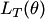is the loss of any linear function of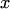. The third term in the bound is 4 times larger than the one of the bound for AAR.

After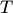steps Ridge Regression minimizes the regularized error: its predictor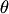achieves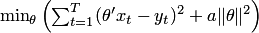, where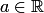.

• Arthur E. Hoerl and Robert W. Kennard. Ridge Regression: biased estimation for nonorthogonal problems. Technometrics, 42:80–86, 2000.
• Katy S. Azoury and Manfred K. Warmuth. Relative loss bounds for on-line density estimation with the exponential family of distributions. Machine Learning, 43:211–246, 2001.
• Fedor Zhdanov and Vladimir Vovk. Competing with Gaussian linear experts. Technical report, arXiv:0910.4683 [cs.LG], arXiv.org e-Print archive, 2009.
• Fedor Zhdanov and Yuri Kalnishkan. An identity for kernel ridge regression. In Proceedings of the 21st International Conference on Algorithmic Learning Theory, 2010.